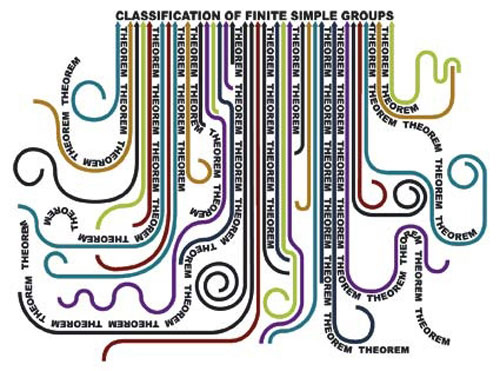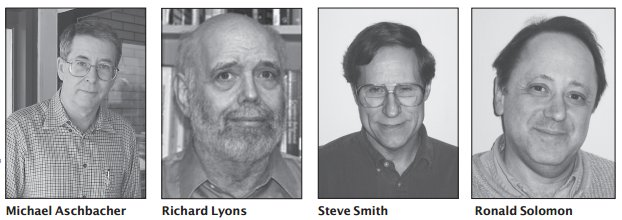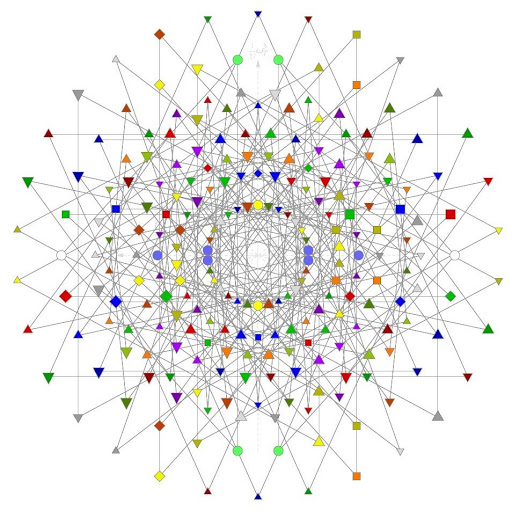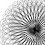### The classification of finite simple groups

A group is a collection of elements that obey to certain rules. For every group we can construct some subgroups, in particular the normal subgroups. Given a group $G$, a subgroup $K$ is normal if, for every element $g \in G$ $gK = Kg$ or in a trivial way if every element $g \in G$ commute with every element $k \in K$.
Now, if the set of the normal subgroups of a nontrivial given group $G$ is constituted only by the trivial group and the group itself, then $G$ is a simple group. And if the group $G$ is finite (the number of the elements of the group is finite), so $G$ is a finite simple group.In order to classify the finite simple groups, Daniel Gorenstein, Ron Solomon and Richard Lyons started in 1980s a program to produce a new and complete proof of the Classification theorem(1):
Every finite simple group is isomorphic to one of the following groups:
• A cyclic group with prime order;
• An alternating group of degree at least 5;
• A simple group of Lie type, including both
• the classical Lie groups, namely the groups of projective special linear, unitary, symplectic, or orthogonal transformations over a finite field;
• the exceptional and twisted groups of Lie type (including the Tits group which is not strictly a group of Lie type).
• The 26 sporadic simple groups.
The work was concluded by Michael Aschbacher and Stephen Smith in 2004: the last chapter of the proof was described in a not so technical paper only by Aschbacher(6) and after in two mathematical monographs(7). The complete classification was finally published in 2011 in the monograph The Classification of Finite Simple Groups: Groups of Characteristic 2 Type by Aschbacher, Lyons, Smith, and Solomon (Mathematical Surveys and Monographs, vol. 172). This work wins the 2012 Leroy P. Steele Prize for Mathematical Exposition(9):In this paper, the authors, who have done foundational work in the classification of finite simple groups, offer to the general mathematical public an articulate and readable exposition of the classification of characteristic 2 type groups.
An interesting and not complete story of the classification theorem is in the Ron Solomon's paper On Finite Simple Groups and Their Classification(4). Reading the paper we can see that just in 1995 the list of all finite simple groups was completed, but there isn't a real complete proof that all groups in the list are finite simple groups. So the Aschbacher and Smith's proof is needed to complete the classification and the atlas of finite representations.
In 2001 Solomon, at the dawn of the conclusion of the work, wrote A brief history of the classification of the finite simple groups(5), and in Applications and others developments section:
Thus the classi cation of all nite groups is completely infeasible. Nevertheless experience shows that most of the nite groups which occur "in nature" - in the broad sense not simply of chemistry and physics, but of number theory, topology, combinatorics, etc. - are "close" either to simple groups(2) or to groups such as dihedral groups, Heisenberg groups, etc., which arise naturally in the study of simple groups. And so both the methodologies and the database of information generated in the course of the Classi cation Project remain of vital importance to the resolution of questions arising in other disciplines.
The story could indeed influence other disciplines, for example physics. Indeed one of the finite simple group is E8, and I'he just explored connection with physics in The universe and the flowers.Another connection with physics is quotedin the first Solomon's work about some particular sporadic simple group(1): the Monster groups. Following Solomon, Monster groups are connected to quantum field theory, and so I try something about this assertion, finding Our Mathematical Universe: I. How the Monster Group Dictates All of Physics by Franklin Potter(8). In paper Potter try to connect the Monster group with Standard Model. The idea, in principle, is not wrong: the Standard Model presents us a universe composed by some finite families of fermions, leptons and bosons, a finite number of elements, like finite groups. Furthermore the three families are constituted by elementary particles, or in other worlds: particles made by themselves, like simple group. So it would be possible to connect Standard Model with some finite simple group, like a Monster group.
I don't know if the way proposed by Potter is correct or not (I read quickly his paper), but he predict the existence of two new quarks, one at 80 GeV and one at 2600 GeV, and this is a good tool in order to say if Monster Group and Standard Model are connected.
Instead Potter is certain that his hypothesis is true:
In this brief article I have outlined specific connections between the mathematics of the Monster Group and fundamental physics particles and rules. These connections support the three hypotheses ERH, MUH, and CUH(3), so I conclude that the Universe is mathematical and that we live in the only possible one. I await the empirical confirmation by the discovery of the 4th quark family, particularly the b' quark at about 80 GeV. Hopefully, the wait will not be long.

Philosophy of the Classification of Finite Simple Groups
An enormous theorem: the classification of finite simple groups by Richard Elwes
The most important people in calssification program was Daniel Gorenstein. Ho was a leader in the group and you can read a bibliography about his work in classification in the two Solomon's papers that I used for this post(4, 5)

Like the elementary particles of physics, sporadic simple groups were often predicted several years before their existence was confirmed. For example, the Monster was predicted in 1973, but not constructed until 1980.
(2) In this sense we can intend the finite simple groups like the atoms of the group theory.
(3) ERH (External Reality Hypothesis): there exists an external physical reality completely independent of us humans; MUH (Mathematical Universe Hypothesis): our external physical reality is a mathematical structure; CUH (Computable Universe Hypothesis): the mathematical structure that is our external physical reality is defined by computable functions.
(4) Ronald Solomon (1995). On Finite Simple Groups and Their Classification. Notices of the American Mathematical Society 42 (02) (pdf)
(5) Solomon, R. (2001). A brief history of the classification of the finite simple groups Bulletin of the American Mathematical Society, 38 (03), 315-353 DOI: 10.1090/S0273-0979-01-00909-0
(6) Michael Aschbacher (2004). The Status of the Classification of the Finite Simple Groups. Notices of the American Mathematical Society 51 (07) (pdf)
(7) Michael Aschbacher, Stephen Smith (2004). The Classification of Quasithin Groups: I. Structure of Strongly Quasithin $\mathcal{K}$-groups and II. Main Theorems: The Classification of Simple QTKE-groups. Mathematical Surveys and Monographs, vol.111-112
(8) Franklin Potter (2011) .Our Mathematical Universe: I. How the Monster Group Dictates All of Physics. Progress in Physics vol.4 (pdf)
(9) Kehoe, E. (2012). 2012 Steele Prizes Notices of the American Mathematical Society, 59 (04) DOI: 10.1090/noti826

#### 1 comment:

1.Thanks!
I tweet soon as possible!

Markup Key:
- <b>bold</b> = bold
- <i>italic</i> = italic
- <a href="http://www.fieldofscience.com/">FoS</a> = FoS